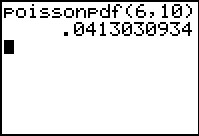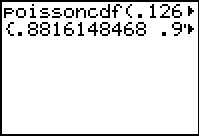# Knowledge Base

## Solution 27230: Performing a Poisson PDF and Poisson CDF on the TI-83 Plus Family of Graphing Calculators.

### How do I use the poissonpdf or possioncdf command on the TI-83 Plus family of graphing calculators?

The command poissonpdf( computes a probability at x for the discrete Poisson distribution with the specified mean μ. Please note that μ must be any real number that is greater than zero; x may be an integer or a list of integers. The syntax is as follows:

poissonpdf(μ, x)

The command poissoncdf( computes a cumulative probability at x for the discrete Poisson distribution with a specified mean μ. Please note that μ must be any real number that is greater than zero; x may be any real number or a list of real numbers. The syntax is as follows:

poissoncdf(μ, x)

Example: Given μ=6 and x=10 find the probability for the discrete Poisson distribution:

1) Press [2nd] [VARS] to access the DISTR menu
2) Press [ALPHA] [APPS] to select B:poissonpdf(
3) Press  [,]   [)] [ENTER] to display the resultExample: Given μ=.126 and x={0,1,2,3} find the probability for the discrete Poisson distribution:

1) Press [2nd] [VARS] to access the DISTR menu
2) Press [ALPHA] [PRGM] to select C:poissoncdf(
3) Press [.]    [,]
4) Press [2nd] [ ( ]  [,]  [,]  [,] 
5) Press [2nd] [ ) ] [ ) ] [ENTER] to display the result